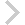FICO Xpress Optimization Examples Repository
 FICO Optimization Community FICO Xpress Optimization HomeSolve a polynomial optimization problem

Description
Create a random polynomial of degree k using the Dot operator and find its minimum using the Nonlinear solver.

Further explanation of this example: 'Xpress Python Reference Manual'

Source Files
By clicking on a file name, a preview is opened at the bottom of this page.

polynomial_opt.py

# Minimize a polynomial constructed with the Dot product
#
# (C) Fair Isaac Corp., 1983-2021

from __future__ import print_function

import xpress as xp
import numpy as np

#
# Generate a random coefficient tensor T of dimension k + 1 and sizes
# n+1 for each dimension except for the first, which is h, then use it
# to create h polynomial constraints. The lhs of each constraint has a
# polynomial of degree k, and not homogeneous as we amend the vector
# of variable with the constant 1. This is accomplished via a single
# dot product.
#

n = 10  # dimension of variable space
h = 3   # number of polynomial constraints
k = 4   # degree of each polynomial

# Vector of n elements: (1, x1, ..., x_{n-1}), declared with NumPy's
# dtype notation for Xpress expressions (to guarantee Xpress
# operations will be used).
x = np.array( + [xp.var(lb=-10, ub=10) for _ in range(n-1)], dtype=xp.npexpr)

sizes = [n]*k  # creates list [n,n,...,n] of k elements

# Operator * before a list translates the list into its
# (unparenthesized) tuple, i.e., the result is a reshape list of
# argument that looks like (h, n, n, ..., n)

T = np.random.random(h * n ** k).reshape(h, *sizes)

print(T)

T2list = [x]*k

compact = xp.Dot(T, *T2list) <= 0

p = xp.problem()